SSC BOARD PAPERS IMPORTANT TOPICS COVERED FOR BOARD EXAM 2024

### Trigonometry Class 9th Mathematics Part Ii MHB Solution

##### Trigonometry Class 9th Mathematics Part Ii MHB Solution

###### Practice Set 8.1
Question 1.

In the Fig. 8.12, ∠R is the right angle of ΔPQR. Write the following ratios.

(i) sin P (ii) cos Q

(iii) tan P (iv) tan Q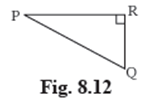For any right-angled triangle,

sinθ = Opposite side Side/Hypotenuse

tanθ = sinθ/cosθ

cotθ = 1/tanθ

secθ = 1/cosθ

cosecθ = 1/sinθ

= Hypotenuse/Opposite side Side

In the given triangle let us understand, the Opposite side and Adjacent sidesides.

So for ∠ P,

Opposite side Side = QR

So, for ∠ Q,

Opposite side Side = PR

In general for the side Opposite side to the 90° angle is the hypotenuse.

So, for Δ PQR, hypotenuse = PQ

(i) sin P = Opposite side Side/Hypotenuse

= QR/PQ

(ii) cos Q = Adjacent sideSide/Hypotenuse

= QR/PQ

(iii) tan P = sinθ/cosθ

= QR/PR

(iv) tan Q = sinθ/cosθ

= PR/QR

Question 2.

In the right angled ΔXYZ, ∠XYZ = 900 and a,b,c are the lengths of the sides as shown in the figure. Write the following ratios,

(i) sin X (ii) tan Z

(iii) cos X (iv) tan X.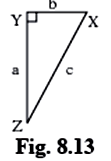For any right-angled triangle,

sinθ = Opposite side Side/Hypotenuse

tanθ = sinθ/cosθ

In the given triangle let us understand, the Opposite side and Adjacent side

So for ∠ X,

Opposite Side = YZ = a

Adjacent Side = XY = b

So for ∠ Z,

Opposite Side = XY = b

Adjacent Side = YZ = a

In general for the side Opposite side to the 90° angle is the hypotenuse.

So for Δ XYZ, hypotenuse = XZ = c

(i) sin X = Opposite side Side/Hypotenuse

= YZ/XZ

a/c

(ii) tan Z = sinθ/cosθ

= XY/YZ

b/a

= XY/XZ

b/c

(iv) tan X = sinθ/cosθ

= YZ/XY

a/b

Question 3.

In right angled ΔLMN, ∠LMN =900, ∠L = 500 and ∠N = 400

write the following ratios.

(i) sin 50° (ii) cos 50°

(iii) tan 40° (iv) cos 40°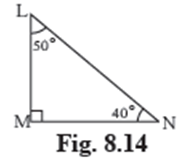For any right-angled triangle,

sinθ = Opposite side Side/Hypotenuse

tanθ = sinθ/cosθ

cotθ = 1/tanθ

secθ = 1/cosθ

cosecθ = 1/sinθ

= Hypotenuse/Opposite side Side

In the given triangle let us understand, the Opposite side and Adjacent sidesides.

So for ∠ 50°,

Opposite side Side = MN

So for ∠ 40°,

Opposite side Side = LM

In general, for the side Opposite side to the 90° angle is the hypotenuse.

So, for Δ LMN, hypotenuse = LN

(i) sin 50° = Opposite side Side/Hypotenuse

= MN/LN

(ii) cos 50° = Adjacent sideSide/Hypotenuse

= LM/LN

(iii) tan 40° = sinθ/cosθ

= LM/MN

(iv) cos 40° = Adjacent sideSide/Hypotenuse

= MN/LN

Question 4.

In the figure 8.15 ∠PQR = 900, ∠PQS = 900, ∠PRQ = α and ∠QPS = θ Write the following trigonometric ratios.

i. sin α, cos α, tan α

ii. sin θ, cos θ, tan θ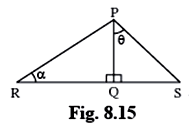For any right-angled triangle,

sinθ = Opposite side Side/Hypotenuse

tanθ = sinθ/cosθ

cotθ = 1/tanθ

secθ = 1/cosθ

cosecθ = 1/sinθ

= Hypotenuse/Opposite side Side

(i) In the given triangle let us understand, the Opposite side and Adjacent sidesides.

So, for Δ PQR,

So, for ∠ α,

Opposite side Side = PQ

In general for the side Opposite side to the 90° angle is the hypotenuse.

So, for Δ PQR, hypotenuse = PR

sin α = Opposite side Side/Hypotenuse

= PQ/PR

= QR/PR

tan α = sinθ/cosθ

= PQ/QR

(ii) In the given triangle let us understand, the Opposite side and Adjacent sidesides.

So for Δ PQS,

So for ∠θ,

Opposite side Side = QS

In general for the side Opposite side to the 90° angle is the hypotenuse.

So for Δ PQS, hypotenuse = PS

sinθ = Opposite side Side/Hypotenuse

= QS/PS

= PQ/PS

tanθ = sinθ/cosθ

= QS/PQ

###### Practice Set 8.2
Question 1.

In the following table, a ratio is given in each column. Find the remaining two ratios in the column and complete the table.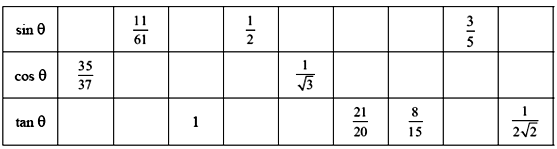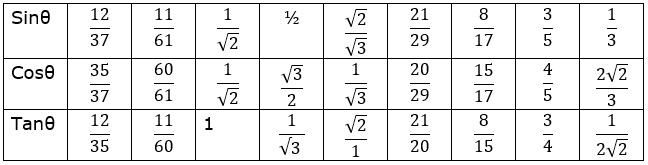For first column: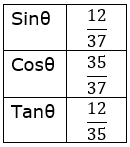cosθ = 35/37

Hypotenuse = 37

By Pythagoras Theorem

Hypotenuse2 = Opposite side2 + Adjacent2

Opposite side2 = Hypotenuse2 - Adjacent2

= 372 - 352

= 1369 – 1225

Opposite side2 = 144

Opposite side = 12

For second column: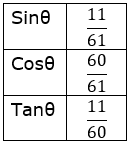Opposite side = 11

Hypotenuse = 61

By Pythagoras Theorem

Hypotenuse2 = Opposite side2 + Adjacent2

Adjacent2 = Hypotenuse2 - Opposite side2

= 612 - 112

= 3721 – 121

For third column: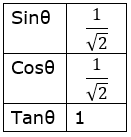Opposite side = 1

By Pythagoras Theorem

Hypotenuse2 = Opposite side2 + Adjacent2

= 1 + 1

Hypotenuse2 = 2

Hypotenuse = √2

For fourth column: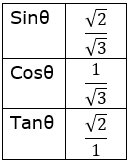Opposite side = 1

Hypotenuse = 2

By Pythagoras Theorem

Hypotenuse2 = Opposite side2 + Adjacent2

Adjacent2 = Hypotenuse2 - Opposite side2

= 22 - 12

= 4 – 1

For fifth column: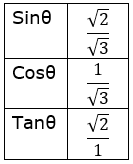Hypotenuse = √3

By Pythagoras Theorem

Hypotenuse2 = Opposite side2 + Adjacent2

Opposite side2 = Hypotenuse2 - Adjacent2

= (√3)2 - 12

= 3 – 1

Opposite side2 = 2

Opposite side = √2

For sixth column: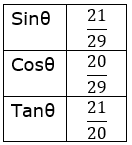Opposite side = 21

By Pythagoras Theorem

Hypotenuse2 = Opposite side2 + Adjacent2

= 212 + 202

Hypotenuse2 = 841

Hypotenuse = 29

For seventh column: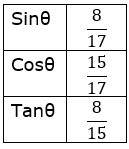Opposite side = 8

By Pythagoras Theorem

Hypotenuse2 = Opposite side2 + Adjacent2

= 82 + 152

Hypotenuse2 = 289

Hypotenuse = 17

For eighth column: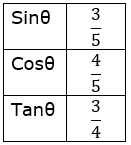Opposite side = 3

Hypotenuse = 5

By Pythagoras Theorem

Hypotenuse2 = Opposite side2 + Adjacent2

Adjacent2 = Hypotenuse2 - Opposite side2

= 52 - 32

= 25 – 9

For ninth column: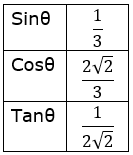Opposite side = 1

By Pythagoras Theorem

Hypotenuse2 = Opposite side2 + Adjacent2

= 12 + (2√2)2

Hypotenuse2 = 9

Hypotenuse = 3

Question 2.

Find the values of –

5sin 300 + 3tan450

We know,

sin 30° = 1/2

tan 45° = 1

⟹ 5sin 30° + 3tan 45°

⟹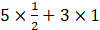⟹ 2.5 + 3

⟹ 5.5

We know,

tan 60° = √3

sin 60° = √3/2

⟹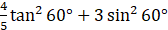⟹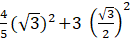⟹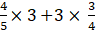⟹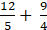⟹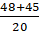= 93/20

Question 4.

Find the values of –

2sin 300 + cos 00 + 3sin 900

We know,

sin 30° = 1/2

cos 0° = 1

sin 90° = 1

⟹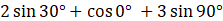⟹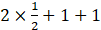⟹ 1 + 1 + 1

= 3

Question 5.

Find the values of –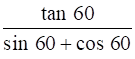We know,

tan 60° = √3

sin 60° = √3/2

cos 60° = 1/2

⟹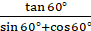⟹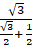⟹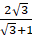Question 6.

Find the values of –

cos2450 + sin2300

We know,

cos 45° = 1/√2

sin 30° = 1/2

⟹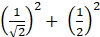⟹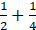⟹Question 7.

Find the values of –

cos 600× cos 300 + sin600 × sin300

We know,

sin 30° = 1/2

sin 60° = √3/2

cos 60° = 1/2

cos 30° = √3/2

⟹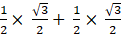⟹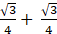⟹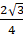⟹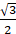Question 8.

If sinθ = 4/5 then find cosθ.

We know,

sinθ = Opposite side/Hypotenuse

Given:

sinθ = 4/5

Opposite side = 4

Hypotenuse = 5

By Pythagoras Theorem

Hypotenuse2 = Opposite side2 + Adjacent2

Adjacent2 = Hypotenuse2 - Opposite side2

= 52 - 42

= 25 – 16

= 9

= 3/5

Question 9.

If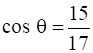then find sinθ

We know,

Hypotenuse = 17

By Pythagoras Theorem

Hypotenuse2 = Opposite side2 + Adjacent2

Opposite side2 = Hypotenuse2 - Adjacent2

= 172 - 152

= 289 – 225

= 64

Opposite side2 = 64

Opposite side = 8

sinθ = Opposite side /Hypotenuse

= 8/17

###### Problem Set 8
Question 1.

Choose the correct alternative answer for following multiple choice questions.

Which of the following statements is true?
A. sin θ = cos(90-θ)

B. cos θ = tan(90-θ)

C. sin θ = tan(90-θ)

D. tan θ = tan(90-θ)

Let us consider the given triangle,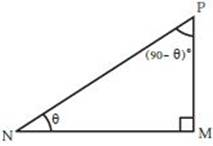In this Δ PMN,

For ∠θ,

Opposite side = PM

For ∠ (90 –θ)

Opposite side = MN

sinθ = Opposite side/Hypotenuse

= PM/PN …………………… (i)

= PM/PN ……………………. (ii)

RHS of equation (i) and (ii) are equal

∴ sinθ = cos (90-θ)

So Option A is correct.

Question 2.

Choose the correct alternative answer for following multiple choice questions.

Which of the following is the value of sin 90°?
A.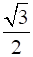B. 0

C.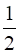D. 1

We know that the value of sin 90° = 1

So option D is correct.

Question 3.

Choose the correct alternative answer for following multiple choice questions.

2tan 450 + cos 450 – sin 450 =?
A. 0

B. 1

C. 2

D. 3

We know that,

tan 45° = 1

We also know that

cos 45° = sin 45°

So,

⟹ 2 × 1 + cos 45° - cos 45°

= 2

So the correct option is C.

Question 4.

Choose the correct alternative answer for following multiple choice questions.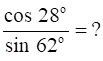A. 2

B. -1

C. 0

D. 1

We know the identity that,

sinθ = cos (90 –θ)

sin 62° = cos (90 – 62)

= cos 28°

Therefore [cos 28°/cos 28°] = 1

So option D is correct.

Question 5.

In right angled ΔTSU, TS = 5, ∠S = 900, SU =12 then find sin T, cos T, tan T. Similarly find sin U, cos U, tan U.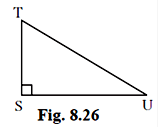Answer: By applying Pythagoras theorem to given triangle we have,
TU2=ST2+SU2
TU2= 52+122
TU2= 25+144
TU2=169
TU=13
Now,
sinT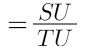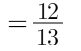cosT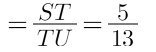tanT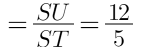Similarly,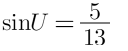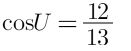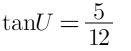Question 6.

In right angled ΔYXZ, ∠X = 900, XZ = 8cm, YZ =17cm, find sin Y, cos Y, tan Y, sin Z, cos Z, tan Z.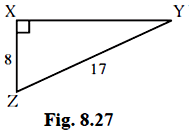For any right-angled triangle,

sinθ = Opposite side /Hypotenuse

tanθ = sinθ/cosθ

cotθ = 1/tanθ

secθ = 1/cosθ

cosecθ = 1/sinθ

= Hypotenuse/Opposite side

In the given triangle let us understand, the Opposite side and Adjacent sides.

So for ∠ Y,

Opposite side = XZ =8

So for ∠ Z,

Opposite side = XY

Adjacent side = XZ = 8

In general for the side Opposite side to the 90° angle is the hypotenuse.

So for Δ TSU,

By Pythagoras Theorem

YZ2 = XZ2 + XY2

XY2 = 172 - 82

= 289 - 64

= 225

XY = 15

(i) sin Y = Opposite side/Hypotenuse

= XZ/YZ

= 8/17

(ii) cos Y = Adjacent side/Hypotenuse

= XY/YZ

= 15/17

(iii) tan Y = sinθ/cosθ

= XZ/XY

= 8/15

(i) sin Z = Opposite side/Hypotenuse

= XY/YZ

= 15/17

(ii) cos Z = Adjacent side/Hypotenuse

= XZ/YZ

= 8/17

(iii) tan Z = sinθ/cosθ

= XZ/XY

= 8/15

Question 7.

In right angled ΔLMN, if ∠N = θ, ∠M = 900, cosθ = 24/25 find sinθ and tanθ Similarly, find (sin2θ) and (cos2θ).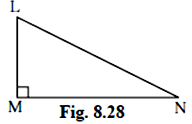Give:

cosθ = 24/25

Hypotenuse = 25

By Pythagoras Theorem

Hypotenuse2 = Opposite side2 + Adjacent2

Opposite side2 = Hypotenuse2 - Adjacent2

= 252 - 242

= 625 – 576

= 49

Opposite side2 = 49

Opposite side = 7

sinθ = Opposite side/Hypotenuse

= 7/25

tanθ = sinθ/cosθ

= 7/24

sin2θ = (7/25)2

= 49/625

cos2θ = (24/25)2

= 576/625

Question 8.

Fill in the blanks.

i.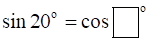ii.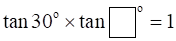iii.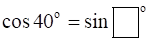i. We know the following identity,

sinθ = cos (90 -θ)

So sin 20° = cos (90 – 20)

∴ sin 20° = cos 70°

ii. We know that,

Let the unknown angle be θ

tan 30° =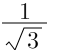tanθ =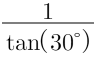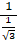tanθ = √3

θ = tan-1(√3)

∴θ = 60°

iii. We know that,

cosθ = sin (90 -θ )

cos 40° = sin (90 – 40)

∴ cos 40° = sin 50°